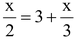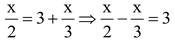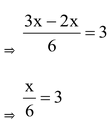# Solution Of Linear Equation In One Variable

## Linear equations in two variable of Class 9

Let ax + b = 0 is one equation then ax + b = 0 ⇒ ax + – b ⇒ x = – b/a is a solution.

question . Solve :Solution: Given⇒ x = 18 Ans.